Email us to get an instant 20% discount on highly effective K-12 Math & English kwizNET Programs!

#### Online Quiz (WorksheetABCD)

Questions Per Quiz = 2 4 6 8 10

### Grade 4 - Mathematics8.9 Types of Fractions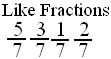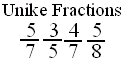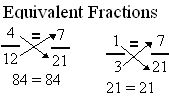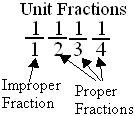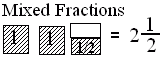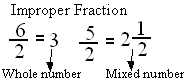Directions: Answer the following questions. Also write at least 5 examples of each type of fractions.
 Q 1: Fractions with different denominators are calledunlike fractionslike fractions Q 2: Fraction with numerator 1 is called a unit fraction. Are all unit fractions proper?NoYes Q 3: Fractions with same denominators are calledunlike fractionslike fractions Q 4: If the numerator is less than the denominator in a fraction, the fraction is called a _______.proper fractionmixed fractionimproper fraction Q 5: When a products obtained by cross multiplication give the same number, then the fractions are ____(or equal).equivalentpropermixed Q 6: A fraction less than 1 is called a proper fraction. A fraction equal to one and more than 1 is called _________.improper fractionmixed fractionproper fraction Q 7: A number with a whole number and a proper fraction is amixed numberproper number Q 8: An improper fraction is sometimes a mixed number or sometimes a whole number.FalseTrue Question 9: This question is available to subscribers only! Question 10: This question is available to subscribers only!

#### Subscription to kwizNET Learning System offers the following benefits:

• Unrestricted access to grade appropriate lessons, quizzes, & printable worksheets
• Instant scoring of online quizzes
• Progress tracking and award certificates to keep your student motivated
• Unlimited practice with auto-generated 'WIZ MATH' quizzes